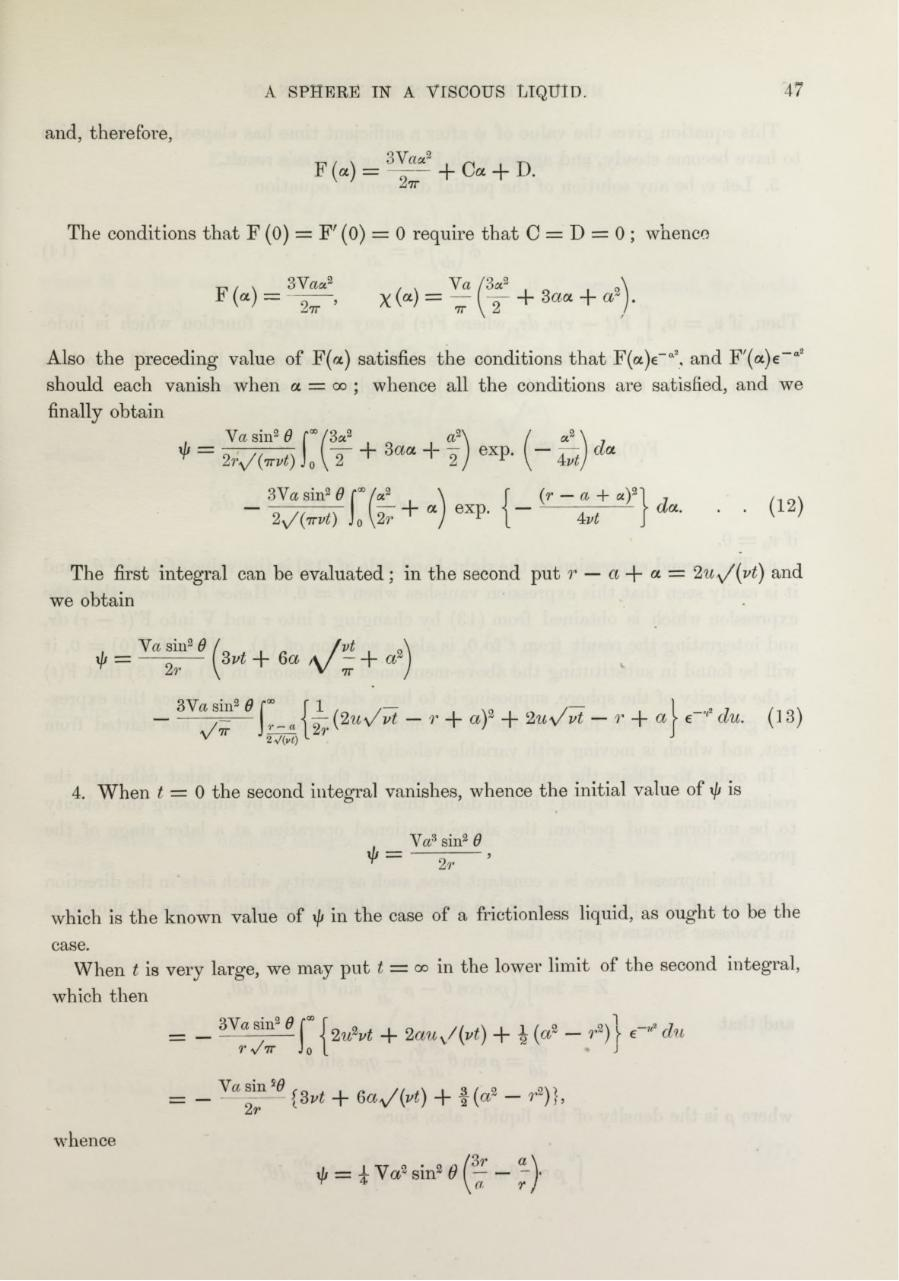# PDF Archive

Easily share your PDF documents with your contacts, on the Web and Social Networks.

## A. B. Basset. On the motion of a sphere in a viscous liquid.pdfPage 1...3 4 56721

#### Text preview

47

A SPHERE IN A VISCOUS LIQUID.

and, therefore,
F (« ) =

3 V««3
-J2tt

Ca -f- D.

The conditions th a t F (0) = ¥ ' (0) = 0 require th a t C = D = 0 ; whence
3V aa2

* » =

~2tt ’

Also the preceding value of F(a) satisfies th e conditions th a t F(a)e-a\ and F '(a)e-a2
should each vanish when a = oo ; whence all th e conditions are satisfied, and we
finally obtain

+ =

V a s in 2 # f°°/3a2

a 2\

2V

2)

^ j U T + 3aa +

/

a2 \

eXP- ( -

3Va sin2 #
2y/

^ —

Yr

\ Vt

/V /

da

(r — a + a)&quot;

C?a.

4^

The first integral can be evaluated; in the second put
we obtain
Vasin2# /

7

(12)

+ a = 2,a v/(i^ ) and

„\

'V

n
~ \ r~

a/

vt

~
r + aY +

4. W hen t = 0 the second integral vanishes, whence the initial value of xfj is
V a3 sin2 #

* =

which is the known value of \/j in the case of a frictionless liquid, as ought to be the
case.
W hen t is very large, we may put t =

00

in the lower lim it of the second integial,

which then
= _
= —
whence

1 2 « V + 2a u K/{yt) + \ (a? - r 2) j e- du
(3vt + 6a^/(vt) + I (a2 — r~)},